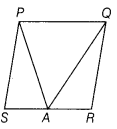# Kumar was having a field in the form of a parallelogram PQRS

Kumar was having a field in the form of a parallelogram PQRS. He divided it into three parts by taking A on RS and joining it to P and Q, such that
(a) one part is exactly half of the given field.
(b) in the remaining two parts , he wants to sow wheat and pulses separately and donates their products to an orphanage.
(i) Show that the above plan is correct.
(ii) Which mathematical concept is used in this problem?
(iii) By donating the product of two parts wheat and pulses to an orphanage, which value is depicted by Kumar?
,

Given, Kumar is having a field in the form of parallelogram PQRS. He marks a point A on RS and joins AP and AQ.(i) Now, the field is divided into three parts of ∆APS, ∆PAQ and ∆QAR. ∆PAQ and parallelogram PQRS are on the same base (PQ) and between the same parallels (PQ || PS).
∴ ar (∆PAQ) = 1/2(parallelogramPQRS) ,…(i)
Hence, the plan of Kumar is correct.
(ii) Areas of parallelograms and triangles.
(iii) The value shown by Kumar is charity.